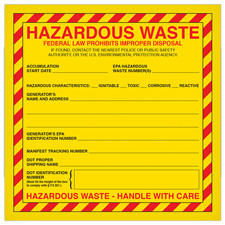MSDSTopics Free Sites FAQ's Regulations Glossary Software Suppliers Books Forum Poll Fun stuff Quiz Store Understand your MSDS with the MS-Demystifier Search ALL our MSDS info

Mole

### Definition

A mole (abbreviation: mol) is an amount of substance that contains as many objects (molecules, particles, ions, cells etc.) as the number of atoms in exactly 12 grams of 12C (carbon).

This number can be determined experimentally to be 6.022 x 1023 (602,200,000,000,000,000,000,000), a number which is called Avogadro's number. The mole is simply a convenient unit for dealing with large numbers just like it is easier to talk about the weight of a ship in tons rather than ounces.

"Mole" has several other possible meanings, of course, but none of these generally applies to Safety Data Sheets.Get your hazardous waste labels from Safety Emporium.

A mole of anything has Avogadro's number of objects in it. So a mole of water (H2O) has 6.022 x 1023 water molecules, a mole of carbon atoms has 6.022 x 1023 carbon atoms and a mole of automobiles (rather unlikely) would have 6.022 x 1023 automobiles.

Clearly, if the objects we are talking about have different masses (weight), then a mole of one substance will also have a different mass than the other. So while a mole of 12C (carbon) atoms will weigh 12 grams, a mole of 197Au (gold) atoms will weigh 197 grams and a mole of sodium chloride (NaCl, table salt) will weigh 58.45 grams.

How were we able to tell you that? Using these terms and definitions:

• The IUPAC definition of atomic mass unit (amu) sets the mass of one 12C atom equal to 12 amu. We can determine the amu experimentally to be 1.66 x 10-24 (0.00000000000000000000000166) grams. Just as Avogadro's number is very large, an amu is very small.

Therefore, the atomic weight of a 12C atom is 12 amu. 14N atom has an atomic weight of 14 amu. You can look these values up in any chemistry textbook or on the web at WebElements.

• The formula weight of a substance is the sum of the atomic weights of each atom in the chemical formula. Therefore, the formula weight of benzene (C6H6) is equal to the mass of six carbon atoms (6 * 12 amu = 72 amu) plus the mass of six hydrogen atoms (6 * 1 amu = 6 amu) = 78 amu. Formula weight is commonly abbreviated FW.
• When the chemical formula equals the molecular formula (see same link), then the formula weight is also called the molecular weight. Therefore, as the chemical and molecular formulas of benzene are both C6H6, the molecular weight of benzene is also 78 amu. The abbreviation commonly used for molecular weight is MW.
• The molar mass is the mass in grams of 1 mole of the substance. Molar masses (in grams) are therefore numerically equal to formula weights (in amu). In the example we've been using, this tells us that because the FW of benzene is 78 amu, then one mole of benzene must weigh 78 grams. Likewise, one mole of water (FW = 18 amu) has a molar mass of 18 grams.

### SDS Relevance

Moles are used to calculate molarity, a unit of concentration most often used for liquid solutions. The formula and molecular weights are also included on Safety Data Sheets in case one needs to calculate a molarity, for example.

Physical property information such as molecular and formula weights is not required on Safety Data Sheets (and is not always applicable), but if this information is on there it will be found in Section 9 (physical and chemical properties) of the SDS.Laboratory operations are a breeze with Ohaus analytical balances from Safety Emporium.

See also: Chemical formula, IUPAC, mass units, molarity.